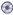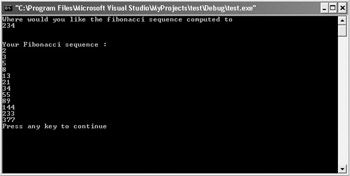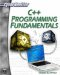# The Fibonacci Sequence

The Fibonacci sequence is an interesting sequence of numbers. It is of particular interest to mathematicians, and is frequently used in computer science to provide a demonstration of recursion. Before we delve into this example of recursion, we should first explore what a Fibonacci number is.

This sequence of numbers was discovered by a mathematician named Fibonacci, thus the name of the sequence. The algorithm used to derive the sequence is quite simple. Examine this series of the first 10 Fibonacci numbers and see if you can derive the algorithm used to produce them.

`1  1  2  3  5  8  13  21  34  55`

Can you guess what is being done? Look closely. If you add the first two numbers, a 1 and a 1, you get the third number, a 2. If you then add the second and third numbers, 1 and 2, you get the fourth number, 3. If you add the third and fourth numbers, 2 and 3, you get the fifth number, 5. This sequence continues infinitely.

The exciting part is where this algorithm pops up. This algorithm is seen throughout nature. The seeds of a sunflower, nodes on the shellfish, a rabbit breeding, and the like all exhibit the Fibonacci sequence. This is why this is so interesting to mathematicians. The sequence can be derived, to any length desired, by a simple recursive function. This is why it is of such interest to computer scientists.

### Example 13.6

Step 1: Enter the following code into a text editor and save it as13-06.cpp.

`#include <iostream> using namespace std; void fibonacci(int, int); int previous = 1; int main() { int stop; cout << "Where would you like the fibonacci "; cout << "sequence computed to \n"; cin >> stop; cout << endl<<endl; cout << "Your Fibonacci sequence : "<<endl; fibonacci(1,stop); return 0;    }// end of main    void fibonacci(int curnum,int max)    { int newnum; newnum = curnum + previous; previous = curnum;  cout << newnum << endl; if(newnum <= max) {  fibonacci(newnum,max); } else {  return ;   }   }`

Step 2: Compile and execute the code. You should see something much like what is depicted in Figure 13.7.Figure 13.7: The Fibonacci sequence.

This example shows you another application for recursive functions. If you carefully examine the code, you see that the user sets the number that will be the cut-off point. That number and the current number being computed are passed to the Fibonacci function. As long as the cut-off has not been reached, the Fibonacci function will continue to call itself repeatedly, each time printing out the current number in the sequence. You can use the program you just saw in the previous example to compute the Fibonacci sequence as far out as you like.C++ Programming Fundamentals (Cyberrookies)
ISBN: 1584502371
EAN: 2147483647
Year: 2005
Pages: 197
Authors: Chuck Easttom